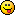# Math Is Fun Forum

Discussion about math, puzzles, games and fun.   Useful symbols: ÷ × ½ √ ∞ ≠ ≤ ≥ ≈ ⇒ ± ∈ Δ θ ∴ ∑ ∫  π  -¹ ² ³ °

You are not logged in.

## #1 2021-05-12 19:08:37

mathland
Member
Registered: 2021-03-25
Posts: 444

### Geometry For You

59. Write the area A of a square as a function
of its perimeter P.

60. Write the area A of a circle as a function
of its circumference C.

61. Path of a Ball You throw a baseball to a child
25 feet away. The height y (in feet) of the baseball is
given by y = − (x^2)/10 x^2 + 3x + 6 where x is the horizontal
distance (in feet) from where you threw the ball.
Can the child catch the baseball while holding a baseball glove
at a height of 5 feet?

Enjoy.

Offline

## #2 2021-08-30 18:22:36

XaiDiv
Member
From: Antarctica
Registered: 2021-08-22
Posts: 25

### Re: Geometry For YouHellooooooooooooo

Offline

## #3 2022-02-24 04:59:36

Rastlin
Member
Registered: 2022-02-24
Posts: 1

### Re: Geometry For You

1/4P*2=A

C=2πr.   A=πr^2      A=C/2^2

y= -(25^2)10/25^2+3*25+6
625.         625.   75
6250/625+81
y=91
No, he couldn't dream of catching that ball.

Offline

## #4 2022-04-11 19:34:51

pamshaw
Member
Registered: 2021-12-07
Posts: 21

### Re: Geometry For You

According to the Question
In order to solve the questions, a little background information of angles and Pythagorean theorem is required.

59: Write the area A of a square as a function
of its perimeter P.
Given that the perimeter of the square = P
Therefore, length of one side of the square = P/4
Area of square = x ^2
A = P²/16

60. Write the area A of a circle as a function
of its circumference C.
Given Circumference = C
Therefore, Radius of the circle = C/2π
Area of a circle = π(C/2π)²
A = C²/4π

61. Path of a Ball You throw a baseball to a child
25 feet away. The height y (in feet) of the baseball is
given by y = − (x^2) /10 x^2 + 3x + 6 where x is the horizontal
distance (in feet) from where you threw the ball.
Can the child catch the baseball while holding a baseball glove
at a height of 5 feet?
y= - (25^2) 10/ 25^2 + 3*25 + 6
6250/625+81
Y =91

Also you can find it here: [mytutorsource.hk/blog/properties-of-right-angle-triangle-and-how-to-apply-pythagorean-theorem]

Last edited by pamshaw (2022-04-12 19:56:46)

Offline